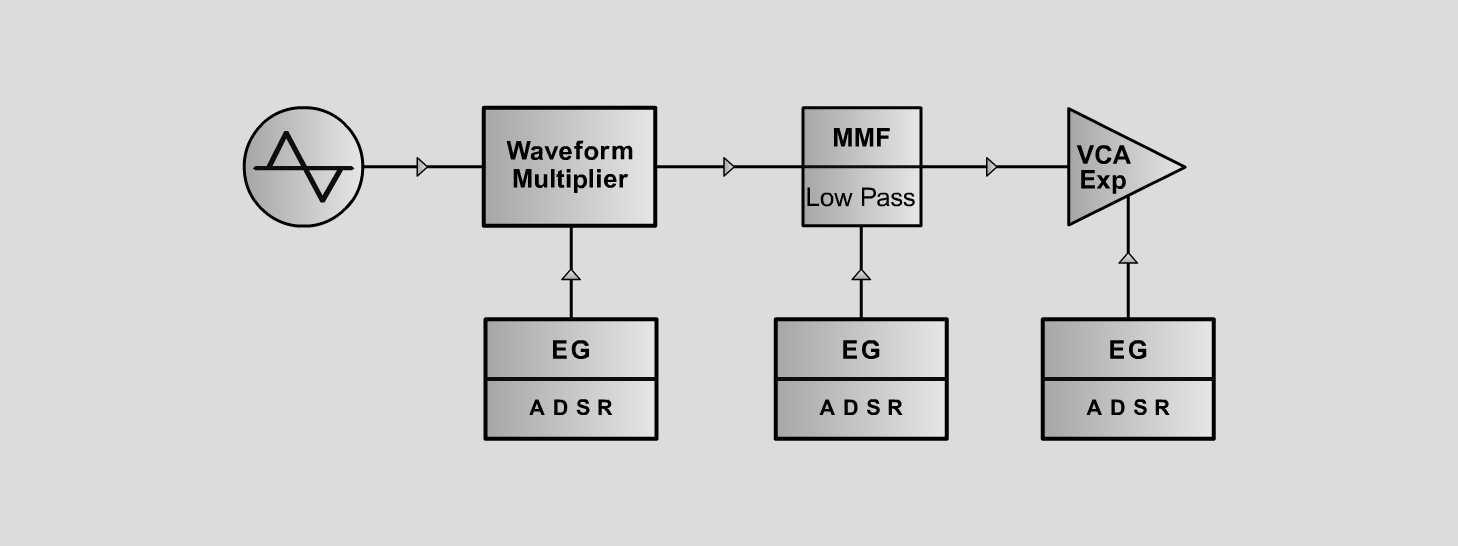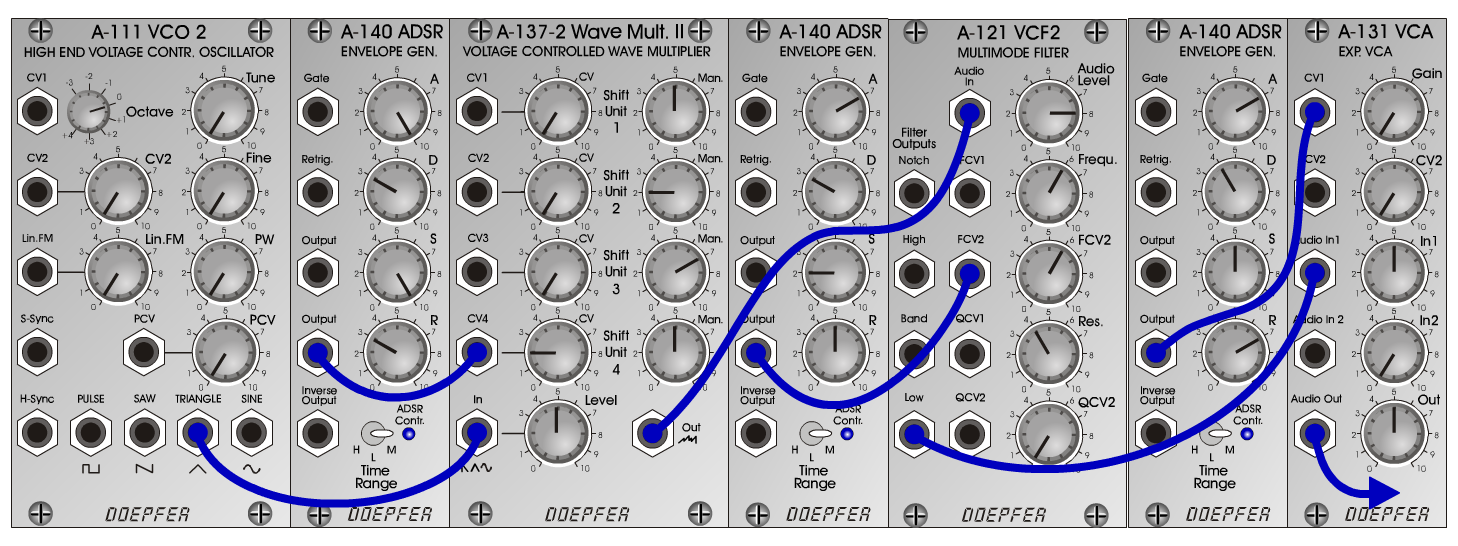Triton PadSynth - P a dD o e p f e r A - 100 Connections: Settings: Triton Pad A-111 (Tri) <=> A-137-2 (In)   A-137-2 (Out) <=> A-121 (Audio In)   A-121 (Low) <=> A-131 (Audio In)   A-140/1 (Output) <=> A-137-2 (CV4)   A-140/2 (Output) <=> A-121 (FCV2)   A-140/3 (Output) <=> A-131 (CV1) A-137-2 (Man1 = 5, Man 2 = 2, Man 3 = 7, Man 4 = 5, CV4 = 2,   Level = 5)   A-121 (Audio Level = 8, Freq = 6, FCV 2 = 6, Res = 4))   A-131 (Gain = 0, Audio In 1 = 5, Audio Out = 5)   A-140/1 (A = 10, D = 3, S = 10, R = 3, Range = M)   A-140/2 (A = 7, D = 3, S = 2, R = 5, Range = M)   A-140/3 (A = 7, D = 4, S = 5, R = 7, Range = M) Josef MuellerSound samples TritonPad (65 Hz) TritonPad (131 Hz) TritonPad (262 Hz)